1596687300

# Lighting the PyTorch tensors

This is a prequel to my previous blog post My first deep learning model using PyTorch. In this tutorial I will be covering basic know how about pytorch tensors.

PyTorch is a python based deep learning framework developed by Facebook’s AI Research. It has gained popularity because of it’s flexibility and speed. It has been integrated with cloud platforms including Amazon’s Sagemaker , Google’s GCP and Azure machine learning service.

## What are tensors?

Tensors are containers that can have data in N-dimensions . A general tensor is represented by N^R where R is rank and N is number of dimensions. From this perspective, a rank-2 tensor (requires N ^2 numbers to describe) is equivalent to an N*N matrix. Scalar can be considered as a rank-0-tensor and vector can be introduced as a rank-1-tensor.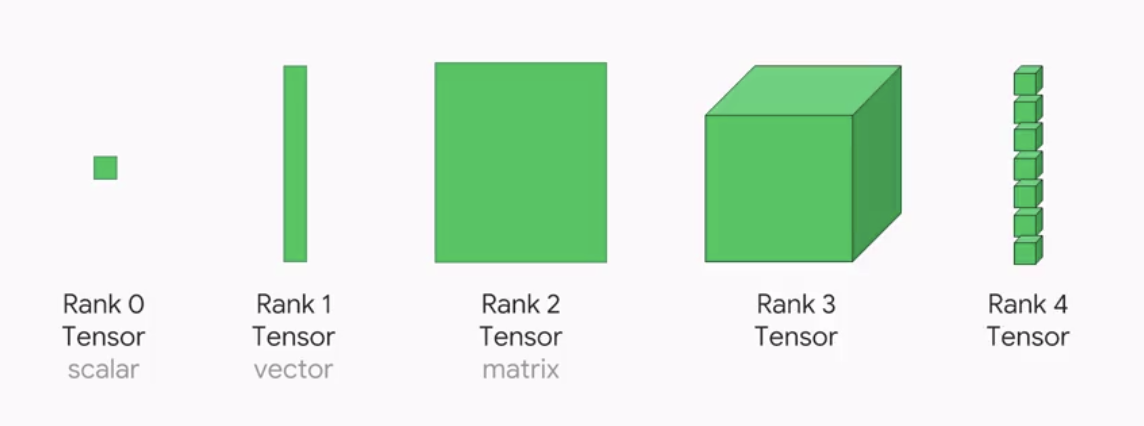## Tensors and NumPy

Tensors are similar to numpy ndarray. Pytorch is targeted as a replacement for numpy to use the power of GPUs. By copying an array to the GPU memory, performance gets improved due to parallel computing.

## 1. Tensor Creation

Although in tensor creation documentation by PyTorch you will find multiple ways to create tensors. Here, I am discussing few popular methods.

**a) torch.tensor **— This is used to create a tensor with pre-existing data.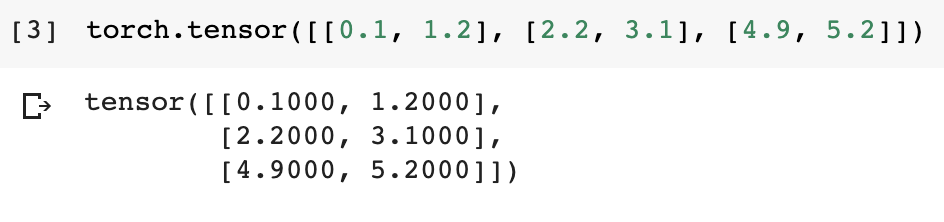**b) torch.zeros **— this method returns a tensor filled with zeroes with shape defined as per the arguments.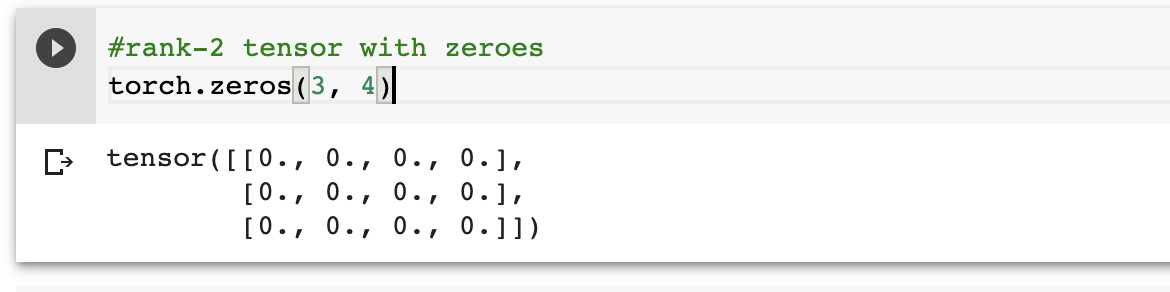c) torch.rand —this method returns a tensor filled with random numbers from a uniform distribution on the interval [0, 1). Similarly for normal distribution torch.randn can be used.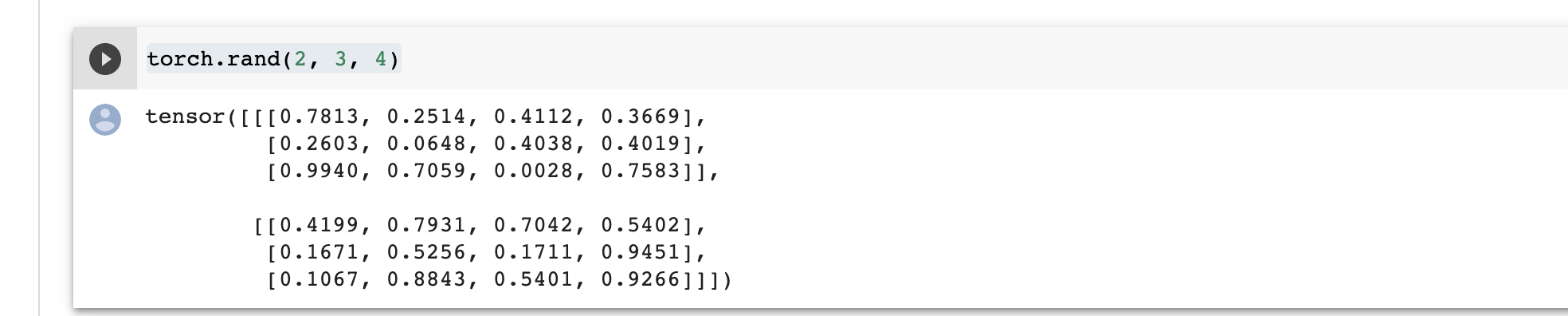**d) torch.from_numpy **— It creates a tensor from numpy ndarray . The returned tensor and numpy ndarraywill share the same memory. Modifications to the tensor will be reflected in the ndarray and vice versa.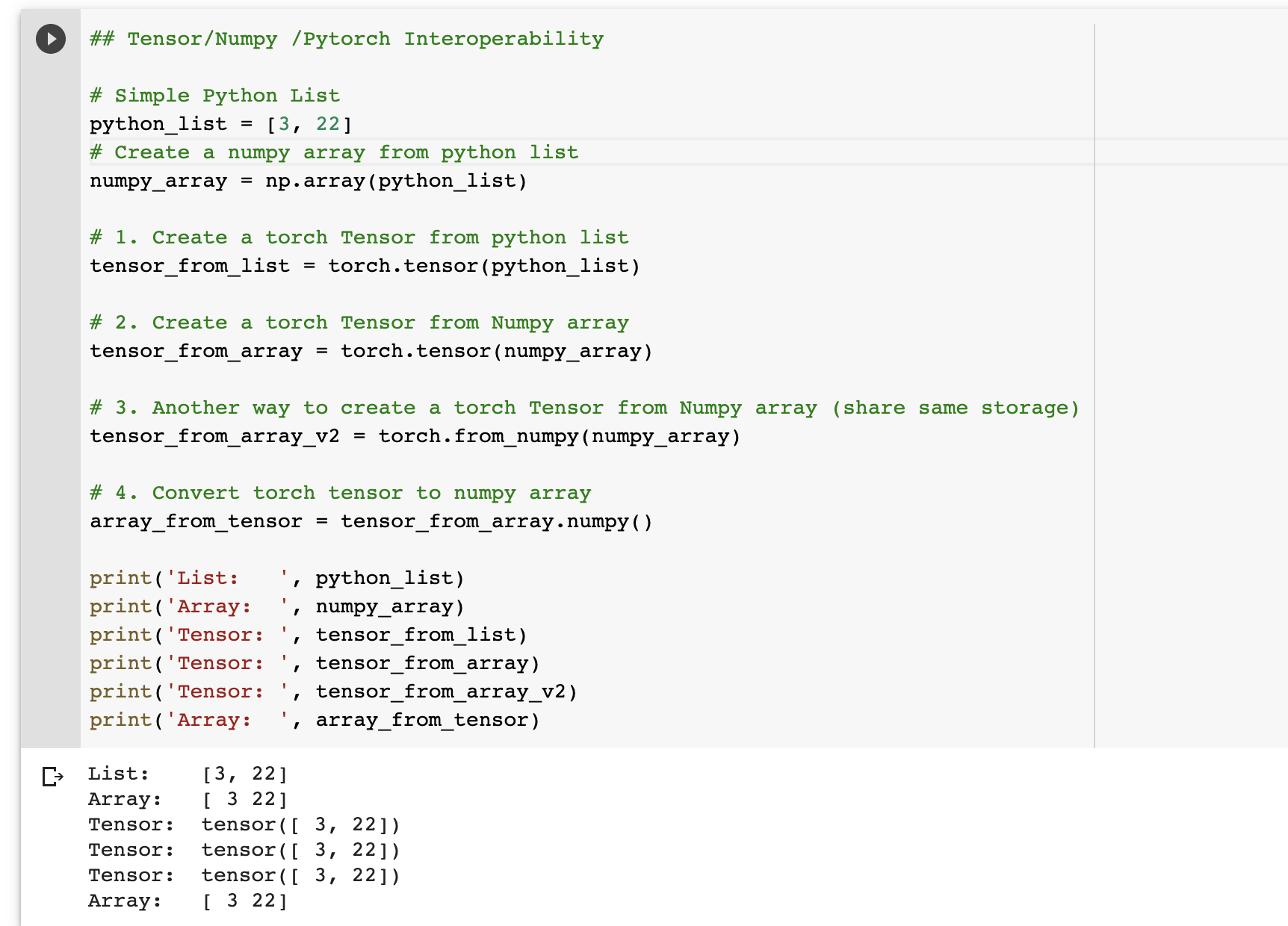Pytorch aims to be an effective library for computations. It avoids memory copying if it can and i.e. the core difference in creating a tensor from numpy array using torch.from_numpy than torch.tensor.

Other methods like torch.randn, torch**.**as_tensor, torch.empty, etc. can be explored for tensor creation too.You can refer herefull list .

## 2. Tensor dimensions

To view shape of a tensor in PyTorch both size() and shape can be used. Shape is an alias to size() and that’s what numpy users would be accustomed to. It is added to match numpy closely.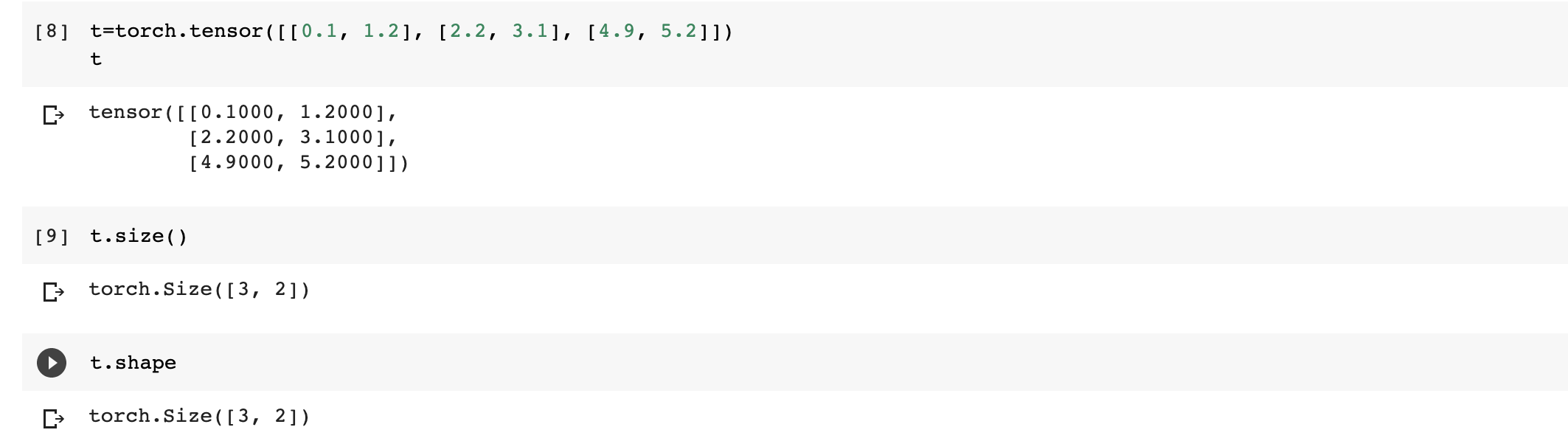You can notice similar outputs for both size() and shape.

## 3. Transforming tensor shapes

To change shape of tensors , view and reshape can be explored. Let’s understand more about both of these methods in detail.

#tensor #autograd #pytorch #deep-learning #neural-networks #deep learning

## Buddha Community1596687300

## Lighting the PyTorch tensors

This is a prequel to my previous blog post My first deep learning model using PyTorch. In this tutorial I will be covering basic know how about pytorch tensors.

PyTorch is a python based deep learning framework developed by Facebook’s AI Research. It has gained popularity because of it’s flexibility and speed. It has been integrated with cloud platforms including Amazon’s Sagemaker , Google’s GCP and Azure machine learning service.

## What are tensors?

Tensors are containers that can have data in N-dimensions . A general tensor is represented by N^R where R is rank and N is number of dimensions. From this perspective, a rank-2 tensor (requires N ^2 numbers to describe) is equivalent to an N*N matrix. Scalar can be considered as a rank-0-tensor and vector can be introduced as a rank-1-tensor.## Tensors and NumPy

Tensors are similar to numpy ndarray. Pytorch is targeted as a replacement for numpy to use the power of GPUs. By copying an array to the GPU memory, performance gets improved due to parallel computing.

## 1. Tensor Creation

Although in tensor creation documentation by PyTorch you will find multiple ways to create tensors. Here, I am discussing few popular methods.

**a) torch.tensor **— This is used to create a tensor with pre-existing data.**b) torch.zeros **— this method returns a tensor filled with zeroes with shape defined as per the arguments.c) torch.rand —this method returns a tensor filled with random numbers from a uniform distribution on the interval [0, 1). Similarly for normal distribution torch.randn can be used.**d) torch.from_numpy **— It creates a tensor from numpy ndarray . The returned tensor and numpy ndarraywill share the same memory. Modifications to the tensor will be reflected in the ndarray and vice versa.Pytorch aims to be an effective library for computations. It avoids memory copying if it can and i.e. the core difference in creating a tensor from numpy array using torch.from_numpy than torch.tensor.

Other methods like torch.randn, torch**.**as_tensor, torch.empty, etc. can be explored for tensor creation too.You can refer herefull list .

## 2. Tensor dimensions

To view shape of a tensor in PyTorch both size() and shape can be used. Shape is an alias to size() and that’s what numpy users would be accustomed to. It is added to match numpy closely.You can notice similar outputs for both size() and shape.

## 3. Transforming tensor shapes

To change shape of tensors , view and reshape can be explored. Let’s understand more about both of these methods in detail.

#tensor #autograd #pytorch #deep-learning #neural-networks #deep learning1595575740

## Introduction to Pytorch and Tensors

I’ve taken up the 6-week course by Jovian.ml on “Deep Learning with PyTorch: Zero to GANs”taught by Aakash N S. In this article (and the following ones), I will share what I’ve learned during the course.

## Intro to PyTorch

Pytorch is an open-source machine learning and deep learning framework developed by Facebook. It is created to process large-scale image analysis, including object detection, recognition and classification and several other tasks. It is written in Python and C++ language. It can be used with other frameworks like Keras etc., to implement complex algorithms.

## Tensors

PyTorch is built on tensors. A PyTorch tensor is an n-dimensional array, similar to NumPy arrays.

## Installing PyTorch

Open the Anaconda terminal, type and run the following command:

conda install pytorch torchvision cudatoolkit=10.2 -c pytorch

## Using Pytorch

Start by importing the PyTorch library into your Jupyter Notebook. I’m importing it as tr (too lazy to type the whole thing). You can just import it as **torch **if you wish.

#tensor #pytorch #deep-learning #machine-learning #framework1595580780

## Tensor operations in PyTorch

PyTorch is a scientific package based on Python, which is used to perform advanced operations using a special datatype known as Tensor. A Tensor is a number, vector, matrix, or multi-dimensional array with regular shape and numbers of the same datatype. PyTorch is an alternative to the NumPy package, which can additionally be used with the power of GPUs. It is also used as a framework for conducting research in deep learning.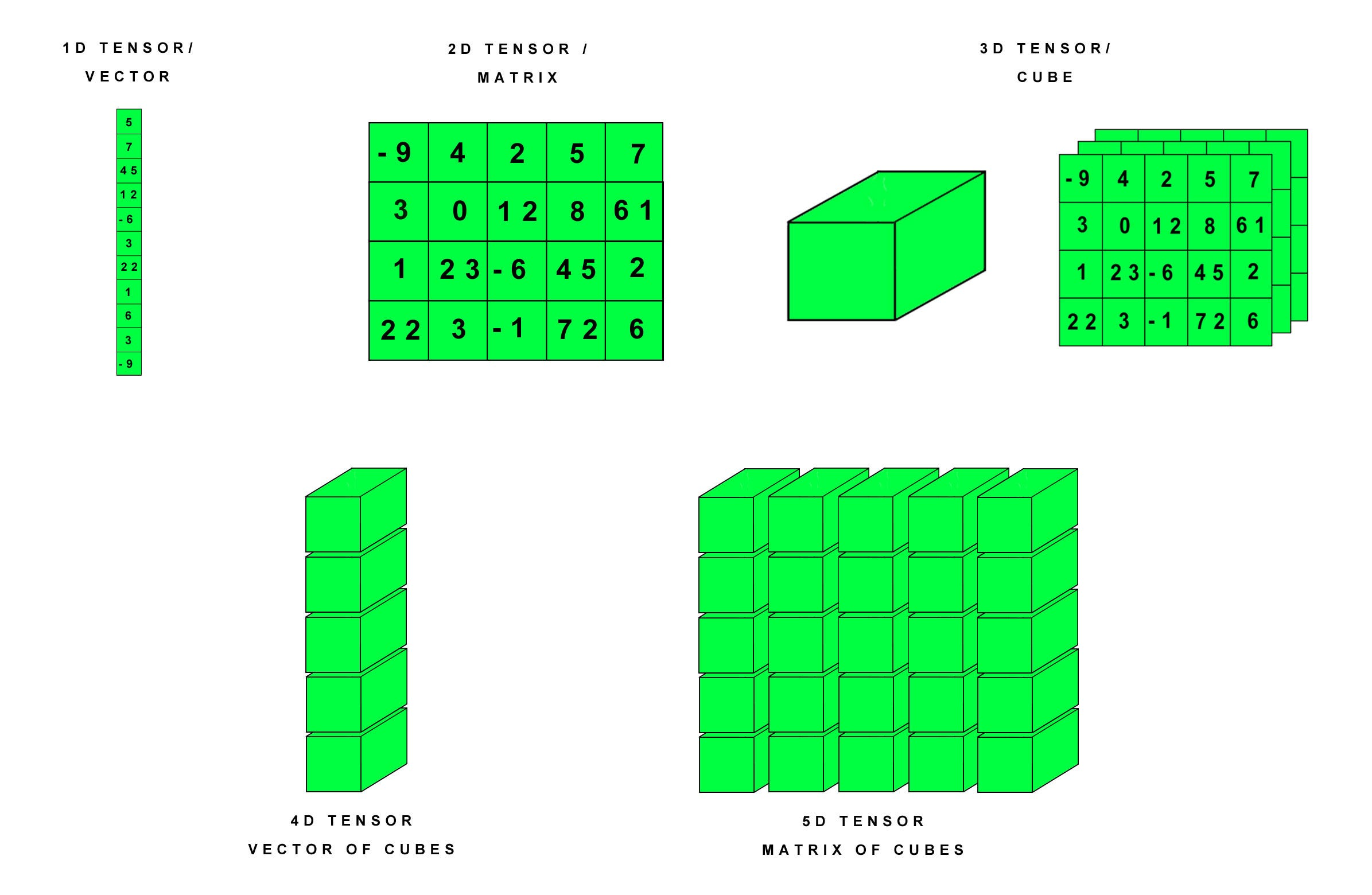Understanding tensors

The 5 operations are:

• expand()
• permute()
• tolist()
• narrow()
• where()

# 1. expand()

The existing tensor is expanded to new dimensions along the dimension with value 1. The tensor can be expanded along any one dimension or multiple dimensions at once. If you do not want to expand the tensor along a particular dimension, you can set its parametric value to -1.

Note: only singleton dimension can be expanded

In this example, the tensor has original dimensions as [1,2,3]. It is expanded to dimensions [2,2,3].

This function can be used to expand existing tensors along singleton dimensions. It only returns a new view and does not allocate new memory. Therefore, it can be used to study how the tensor behaves at larger dimensions.

# 2. permute()

This function returns a view of the tensor with the order of dimensions of original tensor changed according to our choice. For example, if the original dimensions are [1,2,3], we can change it to [3,2,1]. The function takes the required order of dimensions as its parameters.

#deep-learning #tensor #operations #jovian #pytorch #deep learning1591344961

Getting Started with PyTorch
PyTorch is a relatively new and robust deep learning framework, having a dynamic computation graph and supports flexibility. It was designed primarily by Soumith Chinatala, Facebook AI Research.

#tensor #pytorch #machine-learning #programming1600632000

## What is Pytorch ?

Pytorch is a Deep Learning Library Devoloped by Facebook. it can be used for various purposes such as Natural Language Processing , Computer Vision, etc

## Prerequisites

Python, Numpy, Pandas and Matplotlib

## Tensor Basics

What is a tensor ?

A Tensor is a n-dimensional array of elements. In pytorch, everything is a defined as a tensor.

#pytorch #pytorch-tutorial #pytorch-course #deep-learning-course #deep-learning